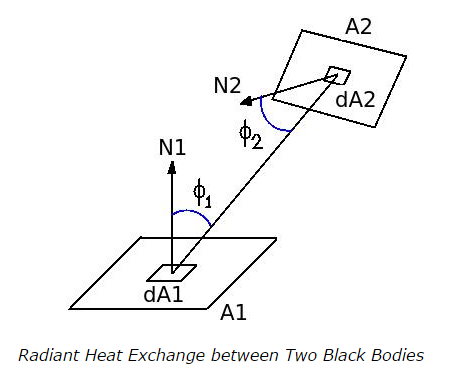# Radiation Heat Exchange between Black Bodies - MCQs

## Radiation Heat Exchange between Black Bodies – MCQs

1. The radiation heat exchange between two bodies depends upon

a. the views of two surfaces with each other
b. the medium between the two bodies
c. emitting and absorbing characteristics of the bodies
d. all of the above

ANSWER: d. all of the above

2. Assume that two black bodies of areas A1 and A2 are placed as shown in figure. dA1 and dA2 are the small area elements of the black bodies. The distance between them is r and the angles made by the normals of the surfaces with the joining line are Φ1 and Φ2 respectively. What is the formula for net heat exchange (Q12)net between A1and A2?

Given that
A1F12 = A2 F21
for the shape factors F12 and F21a. (Q12)net = A1F12 σ (T14 – T24)
b. (Q12)net = A1F12 A2 F21 σ (T14 – T24)
c. (Q12)net = F12 F21 σ (T14 – T24)
d. none of the above

ANSWER: a. (Q12)net = A1F12 σ (T14 – T24)

3. Shape factor is also called as

a. view factor
b. geometry factor
c. configuration factor
d. all of the above

ANSWER: d. all of the above

4. When two bodies of areas A1and A2 are exchanging radiant heat energy, F12 is the shape factor of A1with respect to A2 and F21 is the shape factor of A2 with respect to A1, then what is the correct relation for reciprocating theorem? Assuming A1> A2

a. A1F12 > A2 F21
b. A1F12 < A2 F21
c. A1F12 = A2 F21
d. none of the above

ANSWER: c. A1F12 = A2 F21

5. When F12 is the shape factor of A1with respect to A2 and F21 is the shape factor of A2 with respect to A1and A1= A2, then what is the relation between F12 and F21?

a. F12 > F21
b. F12 < F21
c. F12 = F21
d. none of the above